# IP Corner: Multipliers and DSP Slices

Publish Date: Nov 29, 2018 | 3 Ratings | 3.67 out of 5 | Print | Submit your review

## Overview

The seemingly simple task of multiplying two numbers together can get extremely resource-intensive and complex to implement in digital circuitry. In this edition of IP Corner, learn how FPGA multipliers work, and how to take advantage of the new Virtex-5 DSP48E slices.

### 1. Introduction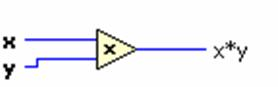Figure 1. Multiply Function in LabVIEW

Multiplication is something that you probably take for granted in field-programmable gate array (FPGA) applications. How difficult could it be to multiply two numbers together? Computers do it all the time, right? The fundamental architecture of a processor-based system (see Figure 2) includes a dedicated calculation engine called the arithmetic logic unit (ALU). The ALU is responsible for all math operations in everything from four-function calculators to Pentium 4 processors.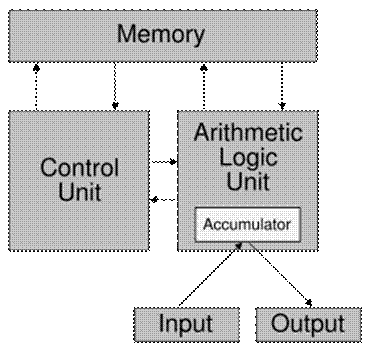Figure 2. Von Neumann Processor Architecture

FPGAs, however, have no ALU, and all operations involve configurable logic blocks that are wired up to define a custom hardware circuit (see Figure 3).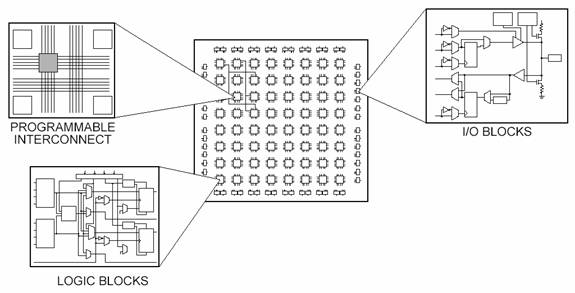Figure 3. An FPGA Composed of Unwired Logic Blocks to Implement a Custom Circuit

You can use logic resources such as flip-flops and look-up tables (LUTs) to perform any type of functionality, but complex math operations like multiplication are extremely resource-intensive. For a frame of reference, refer to Figure 4, a schematic drawing of one way to implement a 4-bit by 4-bit multiplier using combinatorial logic.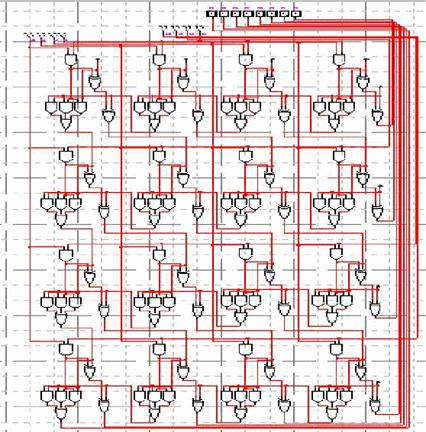Figure 4. Schematic Drawing of a 4-Bit by 4-Bit Multiplier

Now imagine multiplying two 32-bit numbers together, and you end up with more than 2,000 operations for a single multiply. Because of this, many FPGAs have hardwired multiplier circuitry to save on LUT and flip-flop usage in math and signal processing applications. When using a multiply function in the LabVIEW FPGA Module, the compiler tries to use all prebuilt multipliers before building additional ones out of logic resources. Table 1 shows the number of multipliers across various FPGA families.

 Virtex-II 1000 Virtex-II 3000 Spartan-3 1000 Spartan-3 2000 Virtex-5 LX30 Virtex-5 LX50 Virtex-5 LX85 Number of Multipliers 40 96 24 40 32 48 48 Type 18x18 18x18 18x18 18x18 DSP48E Slices DSP48E Slices DSP48E Slices

Table 1. Multiplier Resources for Various FPGAs

Virtex-II and Spartan-3 FPGAs have 18-bit by 18-bit multipliers, whereas the new Virtex-5 family of FPGAs has DSP48E slices with 25-bit by 18-bit multipliers.

### 2. Using Multipliers in LabVIEW FPGA

Consider a 16-bit example in LabVIEW FPGA using an 18-bit by 18-bit multipliers.  The block diagram shown in Figure 5 multiplies two 16-bit numbers together of integer 16 (I16) data type.  In LabVIEW, the default output data type when multiplying two I16 numbers is also I16.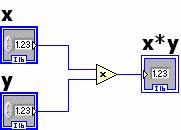Figure 5. Multiplying Two 16-Bit Numbers in LabVIEW FPGA

An 18-bit by 18-bit multiplier has two fixed 18-bit inputs and a fixed 36-bit output to represent all the possible values that can result from multiplying two 18-bit numbers.  When multiplying two 16-bit numbers using one of these multipliers, the corresponding circuit that gets synthesized is shown in Figure 6.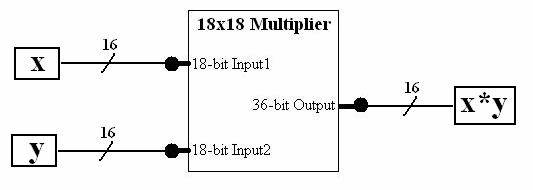Figure 6. 18x18 Multiplier Used to Calculate the Product of Two 16-Bit Numbers

The two 16-bit registers are wired to the inputs of the 18-bit by 18-bit multiplier and a 36-bit value is calculated.  Because the resulting value of x*y in LabVIEW is of I16 data type, only the first 16 bits of the multiplier output are actually used in the block diagram and the remaining 20 bits of the result remain unwired and are essentially lost.  If multiplying the two 16-bit inputs, x and y, produced a value that was larger than 65536 (216) the 16-bit output would overflow and produce incorrect results. This potential for overflow is important to note because it can introduce issues that that are extremely difficult to troubleshoot.

You might have already encountered errors caused by overflow and needed a way to account for the all 32-bit possibilities when multiplying 16-bit numbers. There are two ways to avoid overflow in this example. The first is to convert each number to 32-bit integer values before multiplying, resulting in a full 32-bit output.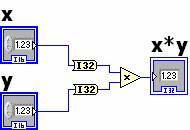Figure 7. Converting to I32 Data Type to Avoid Overflow

Instead of only using the first 16-bits of the multiplier's 36-bit output, this approach will use the first 32-bits, and account for all possible output values.

The second, and more efficient, solution is to use the new fixed-point numeric data type. Even though you might be working with integer numbers and no fractional values, fixed-point math operations are helpful for managing precision and overflow.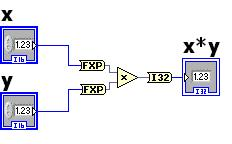Figure 8. Converting to Fixed-Point Data Type to Avoid Overflow and Optimize Usage

By changing the I16 data type to a <±,16,16> fixed-point data type, you can ensure that the multiply operation automatically grows the output data type to  <±,32,32>. This means that you can use all 32 bits of the fixed-point number to represent the integer part of the number. With fixed-point, you also can maximize the inputs of FPGA multipliers with nonstandard bit widths like 18-bit and 25-bit.

For a refresher on how fixed-point math works, please see the  following IP Corner articles:

IP Corner: The LabVIEW Fixed-Point Data Type Part 1 – Fixed-Point 101

IP Corner: The LabVIEW Fixed-Point Data Type Part 2 – Working with Fixed-Point

### 3. Virtex-5 DSP48E Slices

New FPGA architectures have further increased the performance of multipliers and even added other features specifically for digital signal processing (DSP). As mentioned earlier, the new Virtex-5 family of FPGAs offers specialized multipliers called DSP48E slices. In addition to increasing the input sizes from 18x18 to 25x18, these new multipliers include accumulator circuitry to keep a running total of numbers being multiplied. This is also known as a multiply accumulate (MAC) function, and is a common building block for implementing DSP algorithms in hardware. The block diagram in Figure 9 shows how the multiply-accumulate functionality looks in LabVIEW.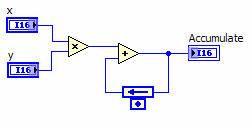Figure 9. Graphical Representation of the Multiply Accumulate Function

However, the block diagram shown in Figure 9 does not actually produce an optimized function that uses the built-in accumulator circuitry. To take full advantage of DSP48E slices in LabVIEW FPGA, the compiler needs lower-level information on how to build an optimized circuit. Because of this, you can now download a high-performance DSP48E MAC function from IPNet that is specifically written for the Virtex-5 resources using the advanced HDL node. This function comes complete with a simulation VI, so you can still verify the functionality of your application without having to compile.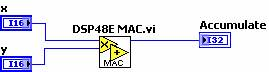Figure 10. DSP48E MAC Function (Available on IPNet)

DSP48E MAC Function

LabVIEW FPGA IPNet

Benefits of Virtex-5 FPGAs

### 4. Summary

Multiplication in FPGAs would be an expensive operation if it weren't for the prebuilt hardware multipliers on many of today’s targets. High-level programming tools such as LabVIEW FPGA make it easy to take advantage of multipliers without having to understand complex hardware design concepts while still providing ways to optimize for performance if necessary. Engineers and scientists can then focus on signal processing algorithms and meeting application requirements with new levels of customization.

IPNet

NI Developer Zone Community

IP Corner addresses issues and presents technical information on LabVIEW FPGA application reusable functionality, also known as FPGA IP. This article series is designed for those interested in learning, testing, or discussing topics to make FPGA designs better and faster through the reuse of IP.

### Ratings

Rate this document